# Skoda cars

There were 16 passenger cars in the parking. It was the 10 blue and 10 Skoda cars. How many are blue Skoda cars in the parking?

Correct result:

n =  4

#### Solution:

$n=10+10-16=4$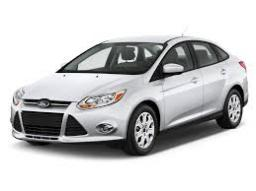We would be very happy if you find an error in the example, spelling mistakes, or inaccuracies, and please send it to us. We thank you!## Next similar math problems:

• The woodenThe wooden block measures 12 cm, 24 cm, and 30 cm. Peter wants to cut it into several identical cubes. At least how many cubes can he get?
• TV competitionIn the competition, 10 contestants answer five questions, one question per round. Anyone who answers correctly will receive as many points as the number of competitors answered incorrectly in that round. One of the contestants after the contest said: We g
• Ten boys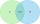Ten boys chose to go to the supermarket. Six boys bought gum and nine boys bought a lollipop. How many boys bought gum and a lollipop?
• Shopping malls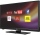The chain of department stores plans to invest up to 24,000 euros in television advertising. All commercials will be placed on a television station where the broadcast of a 30-second spot costs EUR 1,000 and is watched by 14,000 potential customers, durin
• ClassmatesRoman is ranked 12th highest and eleventh lowest pupil. How many classmates does Roman have?
• Derivative problemThe sum of two numbers is 12. Find these numbers if: a) The sum of their third powers is minimal. b) The product of one with the cube of the other is maximal. c) Both are positive and the product of one with the other power of the other is maximal.
• SummandsWe want to split the number 110 into three summands so that the first and the second summand are in the ratio 4: 5, and the third with the first are in ratio 7: 3. Calculate the smallest of the summands.
• The percent 2The percent return rate of a growth fund, income fund, and money market are 10%, 7%, and 5% respectively. Suppose you have 3200 to invest and you want to put twice as much in the growth fund as in the money market to maximize your return. How should you i
• Secret treasureScouts have a tent in the shape of a regular quadrilateral pyramid with a side of the base 4 m and a height of 3 m. Determine the radius r (and height h) of the container so that they can hide the largest possible treasure.
• Hens and pigs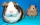Hens and pigs have 46 feet in total. At least how much can heads have?
• Test scoresJo's test scores on the first four 100 point exams are as follows: 96,90,76, and 88. If all exams are worth the same percent, what is the minimum test score necessary on his last exam to earn an A grade in the class (90% or better)?
• Minimum surfaceFind the length, breadth, and height of the cuboid shaped box with a minimum surface area, into which 50 cuboid shaped blocks, each with length, breadth and height equal to 4 cm, 3 cm and 2 cm respectively can be packed.
• Curve and lineThe equation of a curve C is y=2x² -8x+9 and the equation of a line L is x+ y=3 (1) Find the x co-ordinates of the points of intersection of L and C. (2) Show that one of these points is also the stationary point of C?
• Endless lego setThe endless lego set contains only 6, 9, 20 kilograms blocks that can no longer be polished or broken. The workers took them to the gym and immediately started building different buildings. And of course, they wrote down how much the building weighed. The4 m long ladder touches the cube 1mx1m at the wall. How high reach on the wall?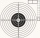The shooter shoots at the target, assuming that the individual shots are independent of each other and the probability of hitting each of them is 0.2. The shooter fires until he hits the target for the first time, then stop firing. (a) What is the most liAn open-topped cylindrical container has a volume of V = 3140 cm3. Find the cylinder dimensions (radius of base r, height v) so that the least material is needed to form the container.